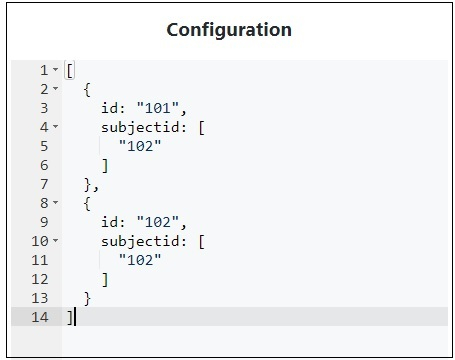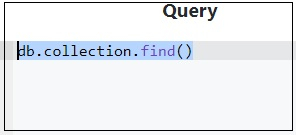# MongoDB query to exclude if id is equal to a document field array value

For this, use $not along with$in. Let us create a collection with documents −

[
{
id: "101",
subjectid: [
"102"
]
},
{
id: "102",
subjectid: [
"102"
]
}
]

Here is the snapshot.Display all documents from a collection with the help of find() method −

db.collection.find()This will produce the following output −

[
{
"_id": ObjectId("5a934e000102030405000000"),
"id": "101",
"subjectid": [
"102"
]
},
{
"_id": ObjectId("5a934e000102030405000001"),
"id": "102",
"subjectid": [
"102"
]
}
]

Following is the query that uses $expr,$not and $in to fetch values excluding matching field array value − db.collection.find({$expr: {
$not:{$in: [
"$id", "$subjectid"
]
}
}
})

This will produce the following output −

[
{
"_id": ObjectId("5a934e000102030405000000"),
"id": "101",
"subjectid": [
"102"
]
}
]